Article published:

Scott Johnson and Hans Walser: Pop-Up Polyhedra. The Mathematical Gazette. Vol. 81, November 1997, 364-380

Pop-up polyhedra

SCOTT JOHNSON and HANS WALSER

Introduction

Once upon a time George Polya showed Jean Pedersen a birthday card sent to him in the form of a pop-up cube. She studied it and subsequently designed a pop-up octahedron (the construction for both of these models is described in ). (Pedersen soon discovered that if her models collapsed it reduced the amount of luggage she had to carry.) With both of these models you can flatten them out, and as soon as you remove your hand, the model will pop up into its original shape.

We describe in this article how to construct some new pop-up models of the platonic solids. We also describe how to construct models that, although they don't pop up, have other tantalizing features. We then tell how these models may be used to illustrate vividly some classical theorems about polyhedra. We give a shorter, more practical, treatment in .

In a final section we give some technical definitions, peculiar to the construction of these particular collapsible polyhedra, along with some very practical information about the actual construction of the models.

The authors wish to thank Jean Pedersen, Santa Clara University, California, for challenging them to build these models and for very helpful criticism during the preparation of the manuscript.

A pop-up model of the edge-jumping cube

In our first model we have to press two opposite edges to flatten the model (Figure 1).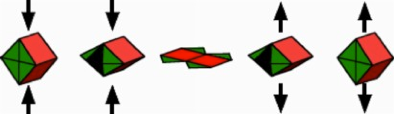F1GURE 1 Pop-up model of the cube

When the opposite edges of this cube are pushed towards each other, the vertical faces will open and we will get the flat situation shown in the centre of Figure 1. If we release the pressure, the rubber band inside the model, attached at two opposite paints labeled R (see Figure 2), will pull together so that the cube pops up. If the model is carefully built, the model will jump into the air.FIGURE 2 One layer

Figure 2 shows one of the two congruent layers of the flattened pop-up cube. A double line indicates a hinge between two pieces of the same layer and a straight line alongside a dotted parallel line indicates a hinge-connection with the congruent second layer. A single straight line indicates the borderline of a piece, which is not connected to any other piece.

Other edge-jumping platonic solids

We learned by experience that models which collapse by pressing and releasing two opposite edges work very well. Figure 3 shows one each of the two layers of the edge-jumping octahedron, the edge-jumping icosahedron and the edge-jumping dodecahedron. The opposite points labeled Ri indicate the attachment of the i-th rubber band.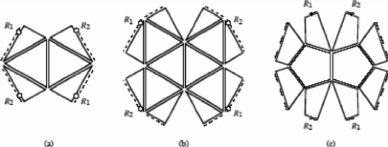FIGURE 3 Edge-jumping octahedron, icosahedron, dodecahedron

The analogous edge-jumping model of the tetrahedron is more sophisticated; this is because opposite edges of a tetrahedron are not parallel. They are in fact orthogonal. In order to construct an edge-jumping tetrahedron we had to invent a way to hinge triangles that touch each other only at a vertex. Figure 4 shows both layers of our model, the top layer seen from outside the tetrahedron, the bottom layer seen from inside. As you would expect, these two layers have a position orthogonal to each other. Each layer consists of two trapezia and two small triangles. The trapezia are hinged by ordinary hinges, but the triangles have only one vertex in common with the trapezia of the same layer. To hinge these triangles at a vertex we need what we call 90°-webs. Corresponding hinges have the same labels. By symmetry we see that these hinges have to lie on the edges of a square. Each layer is composed of two truncated triangles (trapezia) and two small triangles, hinged with 90°-webs.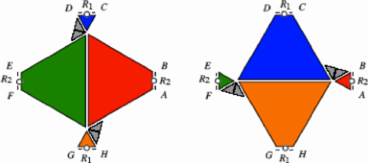FIGURE 4 Edge-jumping tetrahedron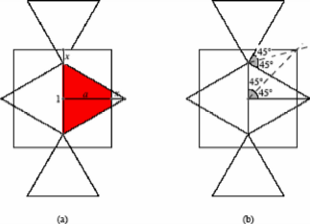F1GURE 5 The height a of the trapezia

The question that needs to be answered, of course, is 'precisely what should be the height of these trapezia?'. To calculate their height a suppose that the edge-length of the tetrahedron is 1. From Figure 5(a) we seehence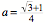. The reader may prove that this height a can be found by using the geometry of Figure 5(b). The pattern of Figure 6 may provide important clues about calculating the value of a by other means.FIGURE 6 A broader view

Models with pivots

The Icosahedron - a face jumper

First, we describe how to build a model of the icosahedron where we have to press together two opposite faces to flatten the model. Let these two opposite faces be the triangle in the middle and the big framing triangle of the Schlegel diagram (A Schlegel diagram of a polyhedron shows what you would 'see' (that is, the connectivity of edges and vertices and the arrangement of faces) if you looked through one face of the model at very close range.) of Figure 7. We divide the set of the 20 triangles of the surface of the icosahedron into two subsets of ten triangles. Each of these two subsets leads to a layer of Figure 8(a).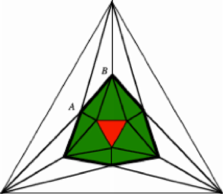FIGURE 7 Schlegel diagram of the icosahedron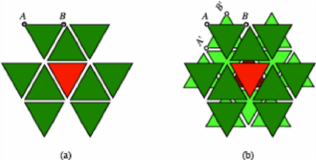F1GURE 8 Layers of the icosahedron

Since two opposite faces of the icosahedron are rotated relative to each other by 60°, the two layers are rotated as well. Thus, superimposing the two layers gives a repeated star-of-David-like situation shown in Figure 8(b). In the popped-up situation, the edges AB and A'B' should coincide. This condition can be achieved by hinging additional hidden triangles to these edges, and connecting these two triangles by brads (brass fasteners) at their centers (see Figure 9, where a brad goes through two layers at point P). This pop-up model will have six of these hidden pivots. The brads at the three pairs of opposite pivots can be used for the attachment of three rubber bands.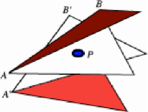FIGURE 9 Pivot P

The cube - a vertex jumper

Now we describe how to build a model of the cube where we have to press together two opposite vertices to flatten the model. This model may be the hardest one to make. We begin by thinking of cutting the cube by the plane perpendicular to the diagonal RS (as shown in Figure10); the intersection of this plane with the cube is a regular hexagon. One half of the surface of the cube    leads to the layout of one layer of our desired model (Figure 11(a)).FIGURE 10 Two opposite vertices R and S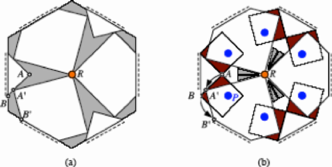FIGURE 11 One layer of our cube with webs and pivots

In the centre R of the layer we need three 30°-webs. The layout of the three right-angled isosceles triangles is such that the 6 borderline hinges lie symmetrically on the edges of a regular hexagon in the flattened situation. In the popped-up situation, the edges AB and A'B' should coincide. This can be achieved by hinging two small squares to each triangle and pivoting them to the big pentagons. The location of those squares and of the pivot is shown in Figure 12.FIGURE 12 The position of the pivot P

The pivot P is situated on the diagonal BC such that the triangle AA'P is equilateral. The triangle BB'P is also equilateral.

The second layer has the same shape, but is rotated by 60°. Every triangle of the top layer is hinged with a pentagon of the bottom layer. Thus we get a model with 12 visible pivots.

Spiral models

In the models we have seen so far the top layer does not turn relative to the bottom layer during the pressing (or collapsing) procedure. We will now show how to build models where each of the two layers rotate as they collapse and expand. These models are not activated by rubber bands, instead they are brought into place by pulled strings.

The spiral icosahedron

In Figure 13 we see an icosahedron as viewed from above (with some of its faces transparent). The top triangle is paler red, the bottom triangle dark red. The six red triangles form a spiral sequence of equilateral triangles running between the top face and the bottom face. Since we have, not counting the top and bottom triangles, 18 triangles remaining, we can, in theory, form three spiral sequences of triangles in this way. We are fortunate that the geometry allows these spirals to fit together to form the icosahedron. This gives us a model with two layers (Figure 14); the two layers are hinged as indicated by labels.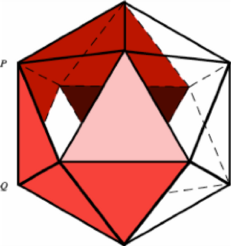F1GURE 13 A spiral sequence of faces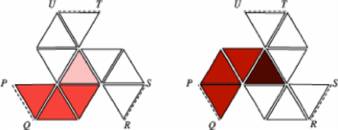FIGURE 14 The two layers

When we first constructed this model, we hoped that, by lifting the central triangle of the top layer, gravity would force the model into the shape of the icosahedron. Surprisingly, we got a right triangular prism with spiral holes on the surface (Figure 15). We had to install a sophisticated system of strings, which pull the vertices of the model into the desired position of the icosahedron (Figure 16).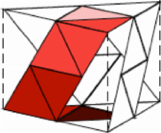FIGURE 15 Right triangular prismFIGURE 16 The three strings of the top layer

In each layer, three strings are attached in the points F1, F2, F3, go through a ring R1, R2, R3 respectively, and exit the model through a central hole H, reinforced by a washer, in the middle of the top face. Here, the three strings of the top layer may be knotted together. A corresponding system of three strings has to be installed in the bottom layer. Starting with the flattened model and pulling at the two opposite knots, the two opposite central faces will turn by 60° and the icosahedron emerges as if by magic.

The spiral dodecahedron

Building the spiral dodecahedron involved us with 'two-way hinges' (which are explained later). These two-way hinges are indicated by a straight line alongside a wavy line in Figure 17. The two-way hinges join the ten bisected lateral pentagonal faces of the dodecahedron.F1GURE 17 The four layers of the spiral dodecahedron

The flattened model consists of four layers: the top layer (Figure 17(a)) connected by an ordinary hinge to the second layer (Figure 17(b)), connected by a two-way hinge to the third layer (Figure 17(c)), connected by an ordinary hinge to the bottom layer (Figure 17(d)). The five pulling strings of the one side are attached at the points F1, F2, F3, F4, F5 on the bottom layer and exit the dodecahedron through the hole H in the centre of the top pentagon and are knotted together. Pulling the two opposite knots forces the two opposite pentagonal faces of the dodecahedron to rotate by 36° and finally the dodecahedron materializes.

The spiral octahedron

Using a method similar to that used in the construction of the spiral icosahedron and dodecahedron, we can build a spiral octahedron (Figure 18). The notation here is the same as for the spiral dodecahedron. Here when we pull the two opposite knots, the opposite triangular faces of the octahedron rotate by 60°.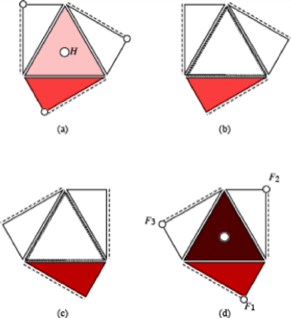FIGURE 18 The spiral octahedron

We challenge the reader to find a corresponding spiral cube - we were not successful in our attempts to do so!

The spiral tetrahedron

Since the tetrahedron has no opposite faces, we decided to be content if we could build a spiral tetrahedron using opposite edges as pressure points. We were successful.

In Figure 19 we see the four layers of this spiral tetrahedron. The two half triangles of the second layer (Figure 19(b)) are not hinged together, but hinged by two-way hinges to the half triangles of the third layer (Figure l9(c)). In the flattened situation, the two opposite edges are parallel. If we pull the model out (holding it by vertices of opposite edges), these two opposite edges rotate, relative to each other, by 90°. This is surprisingly pleasing to the tactile senses.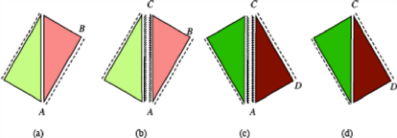FIGURE 19 The spiral tetrahedron

Other models

In all our models, the rotational symmetry with respect to an axis connecting the midpoints of the two opposite constituent parts (Of course the mid-point of a vertex is the vertex itself!) is preserved during the procedures of flattening and releasing. If we do not insist on such symmetries, we can build even simpler pop-up models. For example, Figure 20(a) shows one layer of a very simple model of a pop-up tetrahedron. To flatten this tetrahedron, we simply press together the two adjacent vertices, which are the ends of the open edge. In Figure 20(b) we see one layer of the similar model for the octahedron.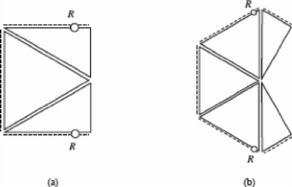FIGURE 20 Pop-up tetrahedron and octahedron

The total angular deficiency

The angular deficiency of a vertex of a polyhedron is the difference between 2π (i.e. 360°) and the sum of the face angles at this vertex. Thus every vertex of the cube has an angular deficiency of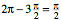, and every vertex of the tetrahedron has an angular deficiency of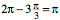. The total angular deficiency (D) of a polyhedron is the sum of the angular deficiencies over all vertices of the polyhedron. The total angular deficiency is a topological invariant; for a polyhedron homeomorphic to the sphere we get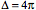. (Check this with some examples!) The total angular deficiency of a polyhedron was first studied by René Descartes.

We may use our pop-up models to illustrate and calculate the total angular deficiency D in some special cases. As an example we take the popup octahedron of Figure 20(b). The angular deficiency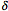of the top vertex is clearly visible (Figure 21(a)). An external angle at a vertex of the 'equator' is half the angular deficiency of this vertex.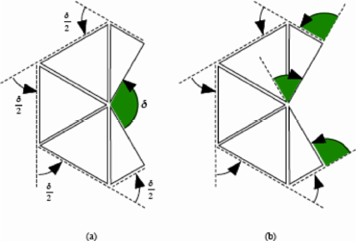F1GURE 21 The angular deficiencyThe angular deficiencyof the top vertex is the algebraic sum of the three shaded external angles shown in Figure 21(b). Notice that the external angle in the middle is negative. Considering also the bottom vertex of the octahedron, we may see that the total angular deficiencyof the octahedron is twice the sum of the external angles of the perimeter of the flattened pop-up octahedron. Since every external angle of a closed polygon is the 'deficiency' (the supplement) of the corresponding internal angle, the sum of the external angles can be considered as the analogue of the total angular            deficiency of a polyhedron. The sum of the external angles of a closed polygon is also a topological invariant. In our case of a simply closed polygon it is 2π. We demonstrate this in familiar terms by thinking of the long nose of Pinocchio. When Pinocchio makes a round trip along the boundary of the polygon, his nose rotates through an angle of precisely 2π (Figure 22).FIGURE 22 The round-trip of Pinocchio

Thus, our models can be used to display a relationship between two topological invariants, one concerning the total angular deficiency of a polygon in the plane, the other concerning the total angular deficiency of a polyhedron in space. The invariant 'pops up' changing the dimension from two to three. Such relationships between an area and its perimeter occur also in the formulae of Stokes, Green and Gauss-Bonnet.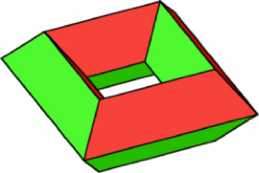FIGURE 23 Torus

Since the total angular deficiency is a topological invariant, it should be interesting to look at a polyhedron of another genus, for instance, at a torus (Figure 23). Figure 24 shows one layer of a pop-up model of a torus. If you want to build this model, use thin cardboard for the inner part, not foamboard. Otherwise you will share with the authors some interesting insights in the topic of non-convexity.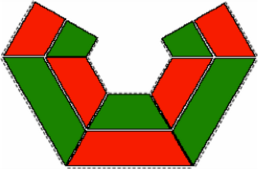FIGURE 24 One layer of the pop-up torus

Now, to examine the total angular deficiency of this torus, we manipulate the perimeter of the flattened model. First we cut the figure into two parts (Figure 25(a)).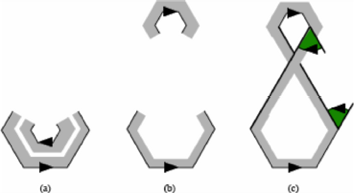FIGURE 25 The total angular deficiency of the torus

Next we move the inner part to another position (Figure 25(b)). Finally, we connect the two parts by straight lines. A straight line does not change anything concerning external angles. Now we have a polygon in the shape of a figure of eight (Figure 25(c)) and such a polygon has zero for the sum of its external angles. This is true because for every positive external angle there is an equivalent negative one (or, think of Pinocchio's nose as he skates this figure of eight!). Hence the total angular deficiency D of our torus is clearly seen to be zero.

Technical definitions and practical hints

We built our models from foamboard (foam, 5mm thick, with a paper covering on both sides), and sometimes from cardboard. For cutting the foamboard we got best results using a surgeon's scalpel or a sharp pocket knife.

Figure 26 shows how we made an ordinary one-way hinge. We first taped the two parts on the inner side with pieces of fiberglass-reinforced tape, then closed the hinge and taped the back of the two parts. These hinges allow motions between 0° and 180° (Figure 27); it is not possible to open more than 180°.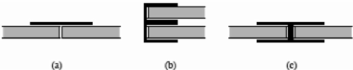FIGURE 26 An ordinary hinge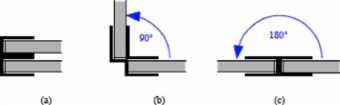FIGURE 27 Range of an ordinary hinge

Some models require hinges with a greater range of motion. These sophisticated two-way hinges require tapes with alternating sticky sides, made from two pieces of one-side-sticky tape, as shown in Figure 28. The two-way hinge is then built by the alternate attachment of such tapes (Figure 29). A two-way hinge allows motions between 0° and 360°.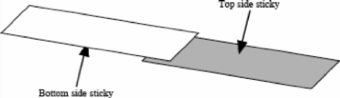FIGURE 28 Tape for a two-way hingeFIGURE 29 Two-way hinge

To affix a rubber band to a hinge, we first reinforce the outside of the hinge by one or two additional reinforced pieces of tape (Figure 30(a)). Then we punch a little hole, pull the rubber band through it (Figure 30(b)) and lock the rubber band with a small wire bolt (wire from paper clips will work, as shown in Figure 30(c)). Finally, we affix the bolt with another piece of tape. For the attachment of the other end of the rubber band, where the rubber band has to be stretched, it is useful to pull the rubber band through the hole with the help of a piece of thread, or a crochet hook.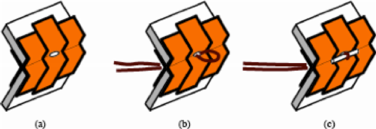FIGURE 30 Attachment of a rubber band

Pivots are made by one (Figure 31(a)) (or two (Figure 31(b))) additional hidden faces made from cardboard (foamboard will be too thick for this purpose). The pivot itself is an ordinary brad.FIGURE 31 Pivots

In some models the pressing procedure separates two or more pieces such that they have no edge, i.e. no hinge, in common, but only a vertex. To construct such 'zero-dimensional hinges' we use webs. We got the inspiration for these zero-dimensional hinges from observing the webs between the claws of a water bird (Figure 32). Our webs are made from cardboard and move inwards when the model pops up. Figure 33 shows the situation where webs are attached to three squares (this situation may occur at a vertex of a cube). We have three 30°-webs, each consisting of two triangles with an angle of 15° and a hinge in betweenFIGURE 32 Web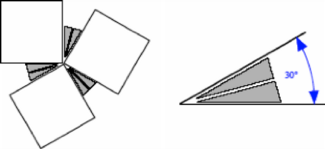FIGURE 33 Web connection

References

1. Peter Hilton and Jean Pedersen, Build your own polyhedra, Addison Wesley, Menlo Park, California, (1994).

2. Scott Johnson and Hans Walser, The pop-up octahedron, Mathematics in School 26 (November 1997).

SCOTT JOHNSON Student at Santa Clara University, Santa Clara, Calilornia 95053, USA

HANS WALSER Santa Clara University, Santa Clara, Calilornia 95053, USA and Mathematik-Departement, Universität Basel, Rheinsprung 21, CH-4051 Basel